# Appendix 1: basic functions and examples

#### 2017-07-03

This vignette corresponds to the latest version of the Appendix 1 of F. Munoz et al. paper.

coalesc() is the key function of the ecolottery package for coalescent-based simulation of local communities. The user can define parameters of community size, migration rate, and a custom function specifying environmental filtering, according to the situation he wants to model. He can also provide relative abundances and species trait values for the regional pool.

set.seed(1)

# Neutral dynamics in species pool and communities

Before simulating communities, we need first to define a reference pool from which immigrants are drawn. In the neutral theory (Hubbell 2001), speciation and extinction events occur randomly irrespective of species properties and drive the composition and species abundances in the reference pool (neutral speciation-drift dynamics). We can define such dynamics by setting the input argument theta in coalesc to a non-null value, and the composition of the pool can be simulated by setting m = 1. The simulated species abundances are then expected to follow a logseries distribution (Hubbell 2001). The fit of simulated pool composition to the logseries distribution can be estimated using the fisherfit function of package vegan.

library(ecolottery)

Jpool <- 25000
logser <- coalesc(Jpool, m = 1, theta = 50)
abund <- abund(logser)

# The expected distribution of abundances in the reference pool is log-series
library(vegan)
## Warning: package 'vegan' was built under R version 3.3.3
## Loading required package: permute
## Loading required package: lattice
## Warning: package 'lattice' was built under R version 3.3.3
## This is vegan 2.4-3
fit <- fisherfit(abund$pool$ab)
freq <- as.numeric(names(fit$fisher)) plot(log(freq), fit$fisher, xlab = "Frequency (log)", ylab = "Species", type = "n")
rect(log(freq - 0.5), 0, log(freq + 0.5), fit$fisher, col = "skyblue") alpha <- fit$estimate
k <- fit$nuisance curve(alpha * k^exp(x)/exp(x), log(0.5), max(log(freq)), col = "red", lwd = 2, add = TRUE)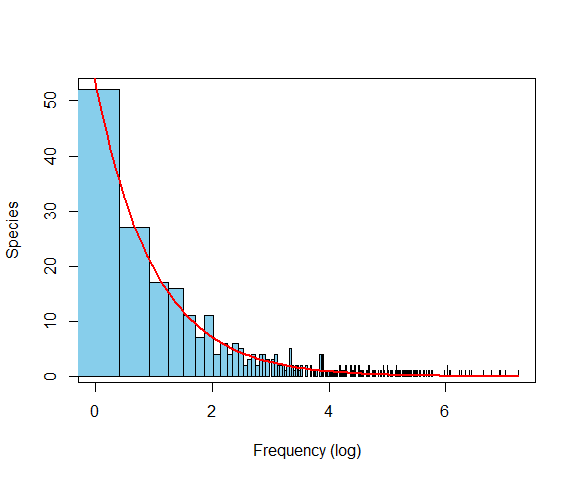We now simulate neutral communities of size J = 500 in which immigrants from the pool establish at rate either m = 0.01 or m = 0.95. In this case, all the species available in the reference pool have the same prospect of immigrating, persisting and reproducing in the local community. We can define such dynamics by setting the input argument filt = NULL in coalesc() (default value). The smaller is m the more local species abundances fluctuate due to stochastic demographic variations. Where m is close to 1, most of the dead individuals are replaced by immigrants, and local species abundances are then more closely related to regional abundances. # Local abundances are averaged over 100 replicate communities J <- 500 m <- 0.01 comm1a <- data.frame() for (i in 1:100) { comm1a <- rbind(comm1a, coalesc(J, m, pool = logser$pool)$com) } comm1a <- list(pool = logser$pool, com = comm1a)

m <- 0.95
comm1b <- data.frame()
for (i in 1:100) {
comm1b <- rbind(comm1b, coalesc(J, m, pool = logser$pool)$com)
}
comm1b <- list(pool = logser$pool, com = comm1b) plot_comm(comm1a, type ="abund", main = "m = 0.01") r_sqa <- summary(lm(abund(comm1a)$pool[rownames(abund(comm1a)$com), "relab"] ~ abund(comm1a)$com$relab)) r_sqa <- signif(r_sqa$r.squared, 2)
legend("bottomright", legend = bquote(R^2 ~ "=" ~. (r_sqa)), bty = "n")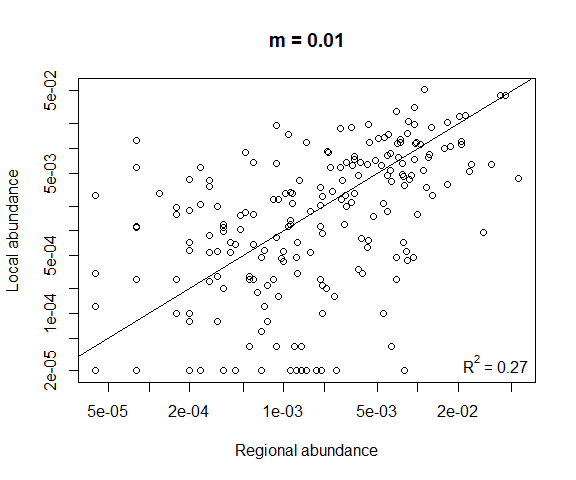plot_comm(comm1b, type = "abund", main = "m = 0.95")
r_sqb <- summary(lm(abund(comm1b)$pool[rownames(abund(comm1b)$com), "relab"] ~
abund(comm1b)$com$relab))
r_sqb <- signif(r_sqb$r.squared, 2) legend("bottomright", legend = bquote(R^2 ~ "=" ~. (r_sqb)), bty = "n")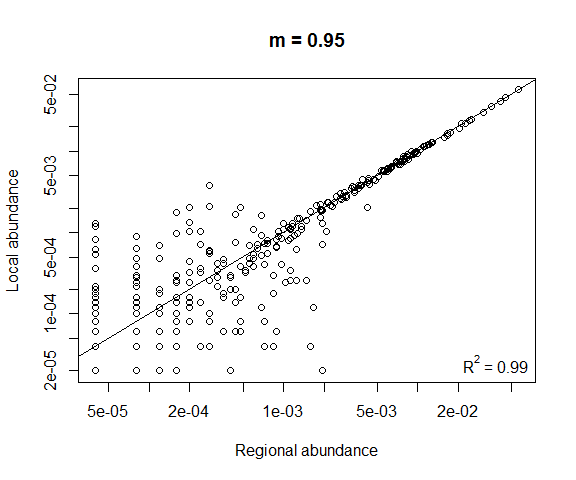The first figure shows the relationship between local and regional species abundances in neutral communities with low immigration rate, and the second the relationship with a high immigration rate. Each point is averaged over 100 communities. # User-defined species pool In the previous case, the composition of the reference pool was simulated depending on the parameter theta of neutral speciation-drift dynamics. In the following example, the user provides a custom species pool including 500 species with equal abundances. Jpool <- 50*J pool <- cbind(1:Jpool,rep(1:500,Jpool/500)) # Generate a neutral community drawn from the pool comm2 <- coalesc(J, m, pool=pool) abund2 <- abund(comm2) summary(abund2$pool$relab) ## Min. 1st Qu. Median Mean 3rd Qu. Max. ## 0.002 0.002 0.002 0.002 0.002 0.002 summary(abund2$com$relab) ## Min. 1st Qu. Median Mean 3rd Qu. Max. ## 0.002000 0.002000 0.002000 0.003145 0.004000 0.010000 hist(abund2$com$relab)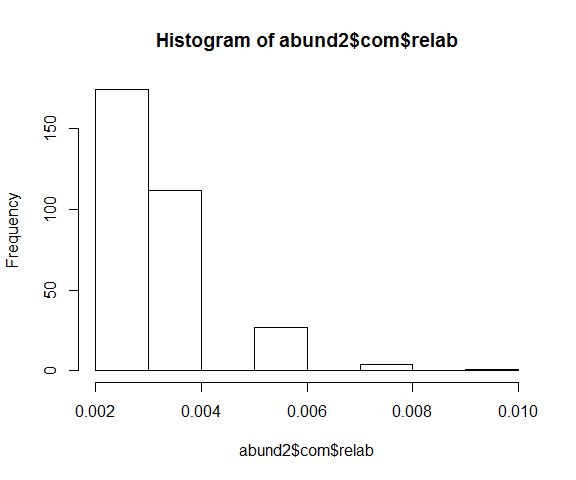While species relative abundances are set equal in the reference pool, local species abundances fluctuate due to migration-drift dynamics. # Trait distribution in communities We can also examine the trait composition of simulated communities. If the user does not provide trait values in the reference pool, the values of a unique trait are randomly assigned to the species of the reference pool following a uniform distribution between 0 and 1. Alternatively, the user can include in pool the values of one or several traits for each individual of the species pool, or provide a separate traits data frame including the values of one or several traits for each species. # With uniform trait values in the species pool pool <- cbind(1:Jpool, rep(1:500, Jpool/500), runif(Jpool)) comm3a <- coalesc(J, m, pool = pool) plot_comm(comm3a, type = "trait")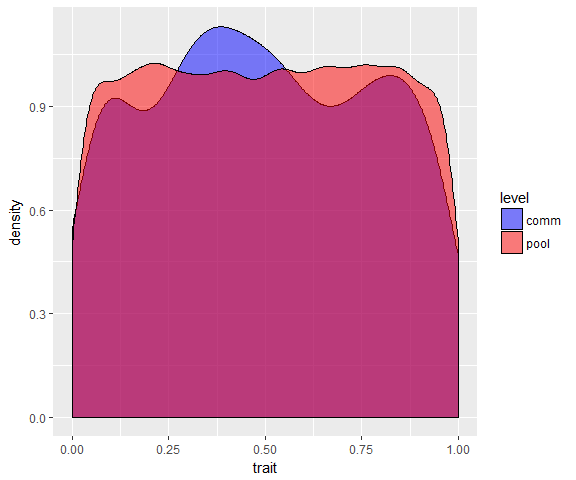# With Gaussian trait values in the species pool pool <- cbind(1:Jpool, rep(1:500, Jpool/500), rnorm(Jpool)) comm3b <- coalesc(J, m, pool = pool) plot_comm(comm3b, type="trait")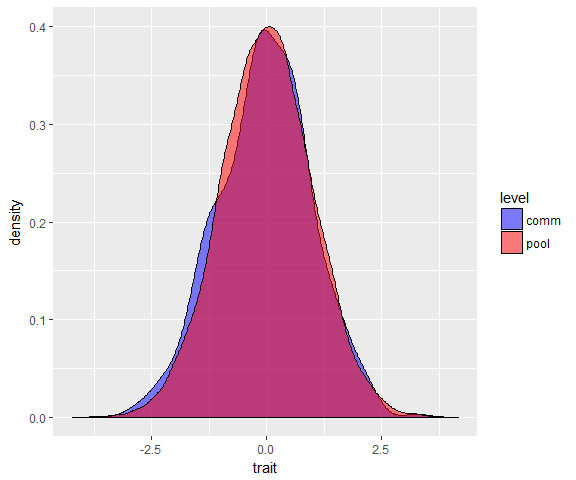plot_comm with type = "trait" here displays the trait distributions in species pool (red) and local community (blue). With high migration probability m = 0.95, the local and regional distributions are quite similar. The user may also simulate a distribution of mean species trait values, and consider additional intraspecific variation of these values around the mean. pool <- cbind(1:Jpool, rep(1:500, Jpool/500), NA) # Distribution of the mean species trait values t.sp <- runif(500) # Gaussian intraspecific variation with standard deviation = 0.01 for(i in 1:500) pool[pool[,2] == i, 3] <- rnorm(sum(pool[,2]==i), mean = t.sp[i], sd = 0.01) comm3c <- coalesc(J, m, pool = pool)  # Environmental filtering The user can provide an environmental filtering function weighting the probability that individuals from the reference pool successfully immigrate in the community, depending on their trait value(s). In the following example, the filtering function is Gaussian with mean t and standard deviation 0.1 (stabilizing filtering, Shipley 2013). sigma <- 0.1 filt_gaussian <- function(t,x) exp(-(x-t)^2/(2*sigma^2)) We simulate a community undergoing stabilizing environmental filtering around t = 0.5. J <- 500; m <- 0.5; comm4a <- coalesc(J, m, filt = function(x) filt_gaussian(0.5, x), pool = pool) plot_comm(comm4a, main = "Stabilizing filtering around t = 0.5")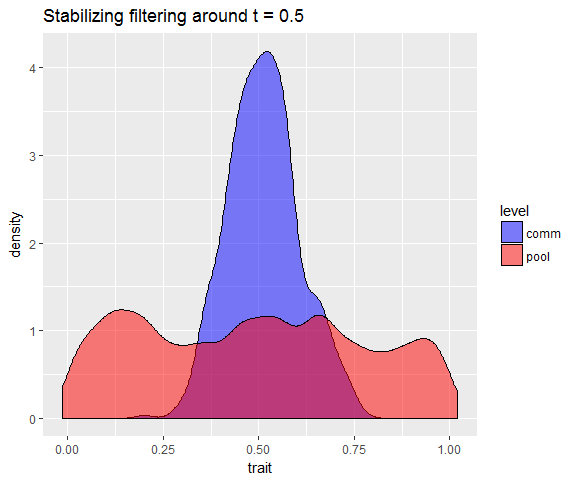We can also simulate stabilizing environmental filtering around t = 0.1 and t = 0.9. J <- 500; m <- 0.5; comm4b <- coalesc(J, m, filt = function(x) filt_gaussian(0.1, x), pool = pool) plot_comm(comm4b, main = "Stabilizing filtering around t = 0.1")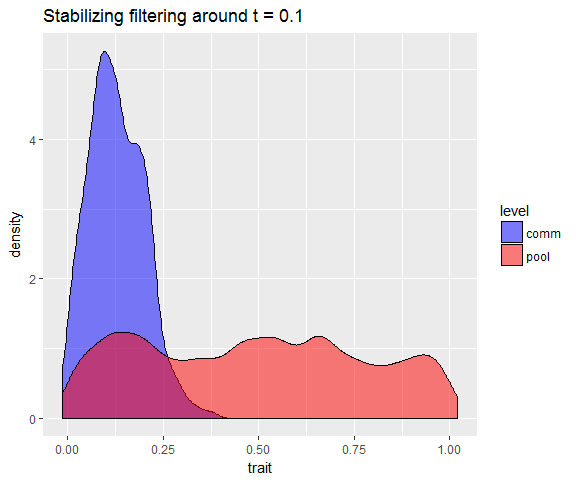comm4c <- coalesc(J, m, filt = function(x) filt_gaussian(0.9, x), pool = pool) plot_comm(comm4c, main = "Stabilizing filtering around t = 0.9")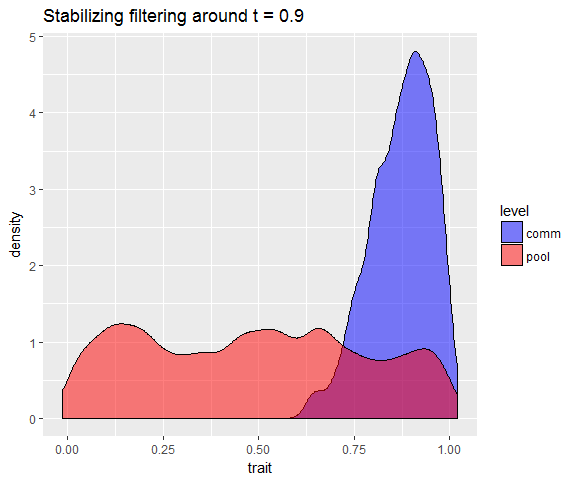When stabilizing filtering operates around different optimal values among communities, we expect corresponding changes in the local mean trait values (Cornwell and Ackerly 2009). mean(comm4b$com[, 3])
##  0.1281551
mean(comm4c$com[, 3]) ##  0.8731013 Different filtering functions can be designed to represent different types of environmental filtering. By analogy with selection regimes in evolutionary theory (Shipley 2013), we can define the outcome of directional and disruptive filtering functions. # Directional environmental filtering toward t = 0 comm4d <- coalesc(J, m, filt = function(x) 1 - min(x,1), pool = pool) plot_comm(comm4d, main = "Directional filtering")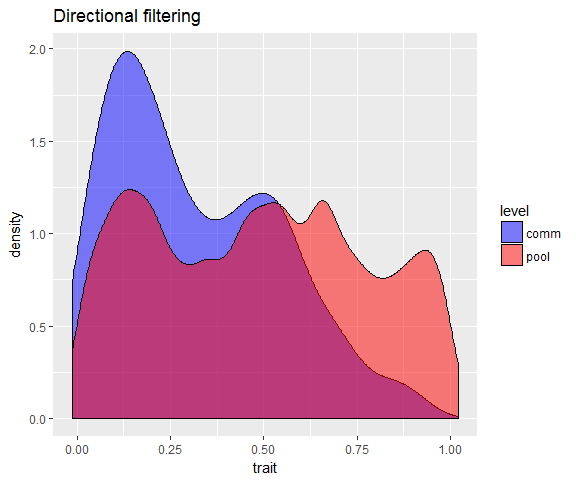# Disruptive environmental filtering around t = 0.5 comm4e <- coalesc(J, m, filt = function(x) abs(0.5 - x), pool = pool) plot_comm(comm4e, main = "Disruptive filtering")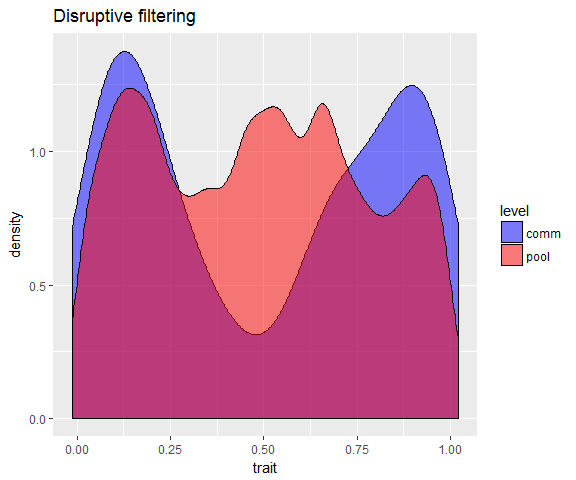Disruptive filtering here represents the greater success of species with trait values away from t = 0.5. It corresponds to a separation of ecological groups in the community, and represents a form of niche differentiation (Vergnon et al. 2009). # Filtering of multiple traits Previous examples represented environmental filtering depending on the values of a single trait. It is also possible to define environmental filtering operating on multiple traits. In the following example, three traits have values uniformly distributed between 0 and 1 in the reference pool, and undergo stabilizing filtering in the local community with distinct optimal values, 0.5, 0.25 and 0.75. # An example with 3 traits traits <- cbind(runif(Jpool), runif(Jpool), runif(Jpool)) filt <- function(x) filt_gaussian(0.5, x)*filt_gaussian(0.25, x)*filt_gaussian(0.75, x) comm5 <- coalesc(J, m, pool = cbind(1:10000, rep(1:100, 100)), filt = filt, traits = traits) The filtering function determines the success of immigrants depending on the combination of their trait values. It entails a correlation between the local species abundances and the species weights given by the filtering function (Shipley et al. 2006). # Relationship between species weight in environmental filtering and local abundance par(mfrow = c(1, 1)) plot(tapply(comm5$com[,3], comm5$com[,2], length) ~ tapply(apply(comm5$com[,3:5], 1, function(x) filt(x)), comm5$com[,2], mean), xlab = "Species filtering weight", ylab = "Species abundance", log = "xy")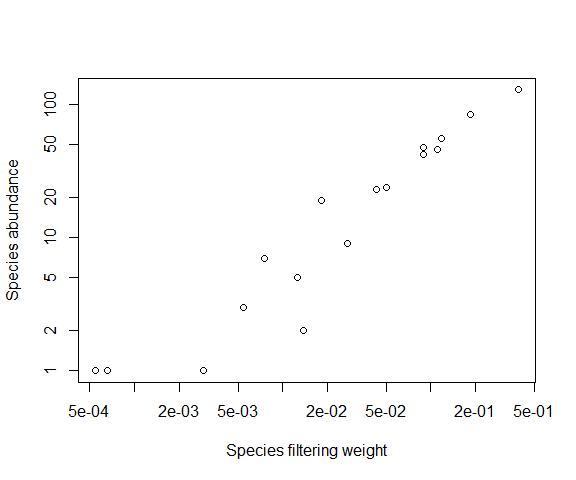# Investigating phylogenetic structure in communities Species trait values are the legacy of evolutionary history. The processes that affect the distribution of trait values in the community can also affect the phylogenetic composition of the community depending, e.g, on the conservatism of traits among close relatives (Mouquet et al. 2012). We can simulate a phylogenetic tree of the species of the reference pool, and the distribution of species trait values under an assumption of niche conservatism (Wiens and Graham 2005). In this case, descendant species can retain characteristics of their ancestors, and the trait values of more closely related species are then more similar than trait values of distantly related species (phylogenetic signal, Blomberg and Garland 2002). library(ape) ## Warning: package 'ape' was built under R version 3.3.3 library(picante) ## Loading required package: nlme ## Warning: package 'nlme' was built under R version 3.3.3 tre <- rcoal(200) Jpool <- 10000 J <- 500 pool <- data.frame(ind=1:Jpool, sp=rep(tre$tip.label,Jpool/50),
tra=rep(NA,Jpool), stringsAsFactors=F)
# Brownian model of trait evolution
t.sp <- rTraitCont(n = 1, phy = tre, model = "BM", sigma = 0.2, root.value = 0.5)
pool$tra <- t.sp[pool$sp]

phylosignal() measures the phylogenetic signal of the simulated trait values in the phylogenetic tree, using the Blomberg’s K statistic (Blomberg and Garland 2002). The p-value is calculated based on the null distribution of K when shuffling species trait values in the phylogeny. A small p-value indicates that trait variation among close relatives is smaller than expected by chance.

phylosignal(t.sp[tre$tip.label], phy = tre) K PIC.variance.obs PIC.variance.rnd.mean PIC.variance.P PIC.variance.Z 2.534453 0.0410402 108.7815 0.001 -1.022508 We then simulate two communities related to this reference pool, one undergoing neutral dynamics, the other undergoing stabilizing environmental filtering. In the first case, any species of the reference pool can perform as well in the local community, and then the phylogenetic structure of the community should not be different from the structure of a random sample with same size from the reference pool. In the second case, environmental filtering limits the range of trait values in the community around an optimal value. Because more closely related species are more likely to have similar trait values in the reference pool, we then expect that the Mean Nearest Taxon Distance (MNTD) among coexisting species of the community is smaller than the average distance in the reference pool (Webb et al. 2002). m <- 1 tab <- array(0, c(2, 200)); colnames(tab) <- tre$tip.label
# First simulation of a neutral community
com <- abund(coalesc(J, m, pool = pool, filt = NULL))$pool tab[1, com$sp] <- com$ab # Second simulation of a community undergoing stabilizing environmental filtering topt <- quantile(t.sp, 0.25) sigma <- 0.01 com <- abund(coalesc(J, m, pool = pool, filt = function(x) exp(-(x - topt)^2/(2*sigma^2))))$com
tab[2, com$sp] <- com$ab

These patterns can be tested against a null model of random sampling in the phylogeny. The standard effect size (SES) quantifies the departure of observed phylogenetic structure from the null distribution.

ses.mntd(tab[, tre$tip.label], cophenetic(tre), null.model = "taxa.labels") Standard Effect Size of Mean Nearest Taxon Distance ntaxa mntd.obs mntd.rand.mean mntd.rand.sd mntd.obs.rank mntd.obs.z mntd.obs.p runs 182 0.0229294 0.0245592 0.0017955 141 -0.907714 0.141 999 20 0.0272001 0.2242011 0.1261642 1 -1.561464 0.001 999 The first community does not depart from random phylogenetic structure, while the second shows phylogenetic structuring, i.e., smaller Mean Nearest Taxon Distance than expected by chance (mntd.obs.p < 0.01). Alternatively, in the absence of niche conservatism in the phylogeny, environmental filtering in community assembly would not result in phylogenetic clustering. To illustrate this case, we shuffle the trait values of species in the reference pool, and simulate a new local community with stabilizing environmental filtering. names(t.sp) <- sample(names(t.sp)) pool$tra <- t.sp[pool$sp] # Again simulating stabilizing environmental filtering com <- abund(coalesc(J, m, pool = pool, filt = function(x) exp(-(x - topt)^2/(2*sigma^2))))$com
tab[2,com$sp] <- com$ab
ses.mntd(tab[,tre$tip.label], cophenetic(tre), null.model = "taxa.labels") Standard Effect Size of Mean Nearest Taxon Distance ntaxa mntd.obs mntd.rand.mean mntd.rand.sd mntd.obs.rank mntd.obs.z mntd.obs.p runs 182 0.0229294 0.0245826 0.0017934 137 -0.9218548 0.137 999 40 0.0685184 0.0951934 0.0329775 108 -0.8088859 0.108 999 In this case, neither the neutral community nor the community with environmental filtering shows phylogenetic clustering. Therefore, when the condition of niche conservatism is not met, the absence of phylogenetic clustering does not mean that environmental filtering does not play (Mouquet et al. 2012). # Forward-in-time simulation The ecolottery package also includes a function forward to perform simulation of community dynamics from an initial composition. As for coalesc, the user can define the composition of the species pool from which immigrants are drawn, and can specify environmental filtering based on one or several traits. We can for instance simulate stabilizing environmental filtering in a way analogous to the previous example with coalesc(). d = 10 represents the number of individuals that die in the community at each time step, prob = 0.5 represents the immigration rate at each time step. The number of simulated time steps is gens = 500. pool <- data.frame(ind=1:Jpool, sp=rep(as.character(1:500),Jpool/500), tra=rep(NA,Jpool), stringsAsFactors=F) t.sp <- data.frame(sp=as.character(1:500), tra = runif(500)) pool$tra <- t.sp[pool$sp,]$tra
# Initial community composed of 500 individuals
initial <- pool[sample(1:Jpool,500),]
# Forward-in-time simulation
sigma <- 0.1
filt_gaussian <- function(t,x) exp(-(x-t)^2/(2*sigma^2))
final.envfilt <- forward(initial = initial, prob = 0.25, d = 10, gens = 500,
pool = pool, filt = function(x) filt_gaussian(0.5,x))
plot_comm(final.envfilt)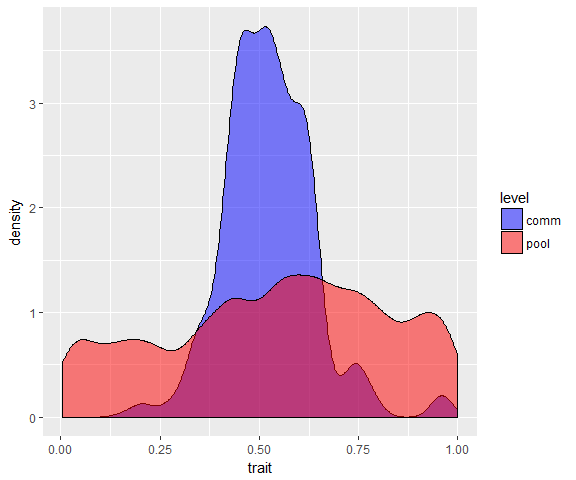A specific advantage of forward() is to simulate the sequence of community assembly events over time. If the birth, death and immigration probabilities depend on community composition at a specific time, the coalescent-based approach may not be appropriate.

In the following example, community dynamics with limiting similarity are simulated. In this case, the probability of individual death depends on the similarity of trait values of each individual to the other individuals of the community.

final.limsim <- forward(initial = initial, prob = 0.25, d = 10, gens = 1000,
pool = pool, keep = F, limit.sim = T, coeff.lim.sim = 1)
plot(final.limsim$dist.t, xlab = "Time", ylab = "Average distance to other individuals") init.dist <- matrix(dist(initial$tra))
diag(init.dist) <- NA
abline(mean(init.dist, na.rm=T), 0, col="red")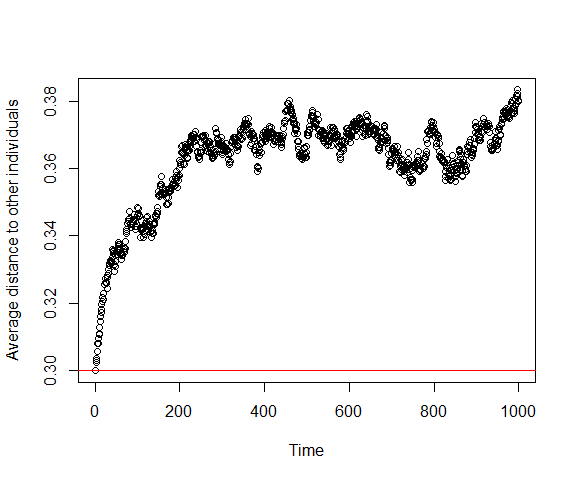plot(final.limsim$sp_t, xlab = "Time", ylab = "Richness") abline(length(unique(initial$sp)), 0, col = "red")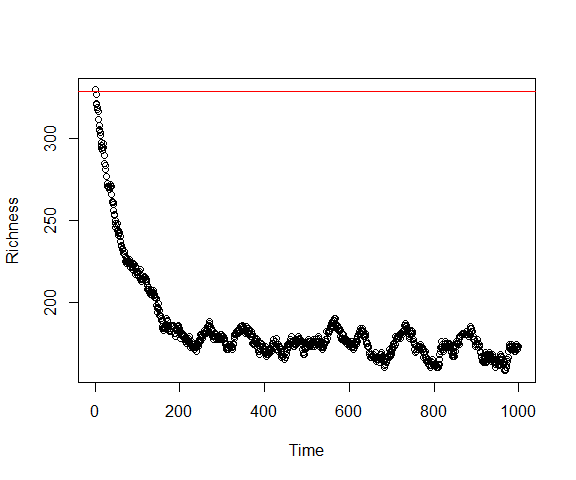The first figure represents the temporal trajectory of the average distance of each individual to the other individuals of the community. The distance fluctuates and increases over time due to the influence of limiting similarity. The second figure shows the variation of richness, basically decreasing due to limiting similarity, until reaching stationarity.